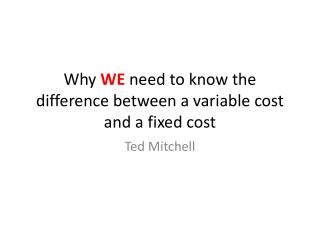DownloadDownload PresentationWhy WE need to know the difference between a variable cost and a fixed cost

# Why WE need to know the difference between a variable cost and a fixed cost

Télécharger la présentation## Why WE need to know the difference between a variable cost and a fixed cost

- - - - - - - - - - - - - - - - - - - - - - - - - - - E N D - - - - - - - - - - - - - - - - - - - - - - - - - - -
##### Presentation Transcript

1. Why WE need to know the difference between a variable cost and a fixed cost Ted Mitchell

2. To Calculate a Breakeven Quantity, BEQ • Breakeven Quantity, BEQ = (Fixed Cost, F) /(Marginal Profit per Unit) • BEQ = F/(Price Tag, P – Variable cost, V) • BEQ = F/(P-V) • Remember it is NOT (Price tag – the average cost per unit) • Price tag is the marginal revenue, P, want to use the marginal cost, V, to establish the marginal profit per unit sold

3. You need to know • 1) What is the marginal profit per unit sold? • Price Tag, P – Variable Cost, V • Marginal Profit Per unit = P-V • 2) The marginal cost is the Same as the Average Cost Per Unit (Unit Cost) • Average Cost per Unit = • (Total Variable Cost, COGS + Total Fixed Cost, F)/ Quantity sold, Q • Average Cost per Unit = (COGS +F)/Q

4. The 4P’s Have a Direct Impact on the Amount Customers Demand for that particular week?

5. The 4P’s can be a Variable Cost or a Fixed Cost

6. The 4P’s can be a Variable Cost or a Fixed Cost

7. What are 3 Ways to set a selling price? • The three C’s of Pricing • 1) Cost Based Based • 2) Competitor Based • 3) Customer (Demand) Based • To know Cost Based Approached you must know the difference between variable cost, V, an average cost and a Fixed Cost, F

8. You need to be able to calculate • a ratio called the Markup on Selling Price, Mp • Markup on Price, Mp = (Price, Tag, P – Variable Cost, V)/Price Tag, P) • Mp = (P-V)/P • Remember it is the variable cost, V, not the average cost per unit • Cost Based Pricing FormulaPrice Tag, P = (Variable cost, V)/(1 – Mp) • P = V/(1-Mp)

9. Example • If you purchase a wagon to be sold in your store with a markup on the selling price of Mp = 60% and it cost you V = \$200 • What is the selling price the customer must pay? • Answer? Mp= (P-V)/P • P = V/(1-Mp) • P = \$200/0.40) = \$500

10. You need to define, identify, and work with • Fixed cost, Variable Cost, average cost per unit • Breakeven Quantity • Markup on Selling Price • Any questions?